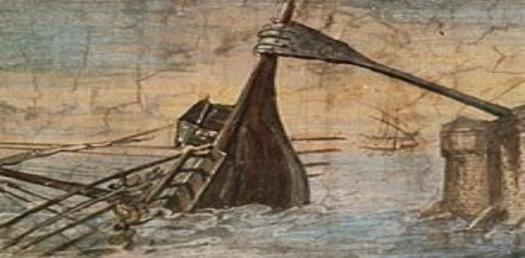# A Quiz About Axiom Archimedes

10 Questions | Total Attempts: 102SettingsAxiom Archimedes or Archimedean property is the property of having no infinitely or infinitely small elements. It was derived from the work of Archimedes, known as the Axiom V of Archimedes, on Spheres and Cylinders. Try to ace this quiz.

Related Topics
• 1.
Who gave the axiom of Archimedes its name because it appears as Axiom V of Archimedes on Spheres and Cylinders?
• A.

Holms

• B.

Otto Stolz

• C.

Ostrowski

• D.

Archimedes

• 2.
Which of these is not a property of ordered field?
• A.

It contains irrational number

• B.

If x is infinitesimal its inverse is also infinite

• C.

It contains rational number

• D.

If x is infinitesimal and r is rational their products are also infinite

• 3.
When a pair of non-zero elements is infinitesimal with respect to the other, what is it called?
• A.

Strowski theorem

• B.

Archimedean

• C.

Non-Archimedean

• D.

Heron's theorem

• 4.
In which of the following is the Archimedean principle very useful?
• A.

Cinematography

• B.

Local field

• C.

Mechanics

• D.

Medicine

• 5.
When was the Archimidean property named The Axiom  Archimedes?
• A.

1970s

• B.

1980s

• C.

1880s

• D.

1870s

• 6.
Which of these statements is the Axiom of Archimedes?
• A.

Let x be any element of K. Then there exists a rational number r such that r > x

• B.

Let x be any element of K. Then there exists a natural number n such that n = x

• C.

Let x be any element of K. Then there exists a natural number n such that n < x

• D.

Let x be any element of K. Then there exists a natural number n such that n > x

• 7.
What does K represent in Axiom of Archimedes?
• A.

Ordered field

• B.

Strowski determinant

• C.

Axiom constant

• D.

Archimedes constant

• 8.
Which structure holds the Archimedean principle?
• A.

Index structure

• B.

Strowski Structure

• C.

Algebraic structure

• D.

Rational number structures

• 9.
Which property must be non-Archimedean in the context of ordered field?
• A.

Irrational function

• B.

Rational function

• C.

Real number function

• D.

Strowski constant

• 10.
Which of the following is not equivalent characterizations of Archimedean fields?
• A.

The natural numbers are cofinal in K

• B.

For any x in K the set of integers greater than x has a least element

• C.

Zero is the infimum of a set

• D.

Every nonempty open interval of K contains a natural number